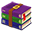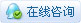9688浏览

# [ESP8266/ESP32] 零基础向：用Beetle ESP32 C3点亮TFT屏幕本帖最后由 伊娃老师 于 2023-5-27 14:48 编辑 很多好玩的项目离不开一个能显示各种内容的显示屏，我为了在esp32上点亮一个TFT屏幕，折腾了10小时才成功，为了让大家不要经历我这种苦难，我把踩过的坑总结给大家，零基础也能点亮TFT。 【准备器材】 1. 主控板： Beetle ESP32 C3：相比一般的ESP32更加小巧，也能直接插在面包板连接TFT 或者 一般的ESP32 2. TFT屏幕：我使用的是ST7735s的驱动，分享的内容不限此驱动，适用大部分TFT 3. 其他：面包板、面包线 4. 库文件：TFT_eSPI.zip (5.91 MB, 下载次数: 54) 2023-5-27 14:45 上传 点击文件名下载附件【点亮TFT步骤】 1. 在Arduino设置ESP32环境 管理器地址： https://dl.espressif.com/dl/package_esp32_index.json https://github.com/Bodmer/TFT_eSPI 2. 安装TFT_eSPI库 3. 连线 脚位对应： TFT_SCLK：对应SCL TFT_MOSI：对应SDA TFT_RST        ：对应RES    TFT_DC        ：对应DC             TFT_CS        ：对应CS TFT_BL        ：对应BL 4. 更改User_setup.h中的驱动与引脚设置 5. 上传代码 6. 点亮TFT 【测试代码】 .hljs {margin: 10px 0px;}.hljs ol,.hljs li{list-style: decimal;}.hljs li {margin-left: 0em;}``````#include   // 引入 TFT_eSPI 库 TFT_eSPI tft;  // 创建 TFT_eSPI 对象 void setup() {   tft.init();  // 初始化 TFT 屏幕   tft.setRotation(0));           // 调整屏幕方向，根据需要选择合适的值（0、1、2、3）   tft.fillScreen(TFT_BLACK);    // 设置屏幕背景颜色为黑色   tft.setTextSize(1);           // 设置文本大小   tft.setTextColor(TFT_WHITE);  // 设置文本颜色为白色 } void loop() {   tft.fillScreen(TFT_BLACK);  // 清空屏幕，将屏幕填充为黑色   // 绘制不同图形   // 绘制一个像素点，参数：x 坐标，y 坐标，颜色   tft.drawPixel(5, 5, TFT_RED);   // 绘制一条直线，参数：起点的 x 坐标，起点的 y 坐标，终点的 x 坐标，终点的 y 坐标，颜色   tft.drawLine(10, 20, 60, 20, TFT_GREEN);   // 第一行：矩形   // 绘制一个矩形边框，参数：左上角的 x 坐标，左上角的 y 坐标，矩形的宽度，矩形的高度，颜色   tft.drawRect(10, 40, 15, 10, TFT_BLUE);   // 绘制一个矩形填充，参数：左上角的 x 坐标，左上角的 y 坐标，矩形的宽度，矩形的高度，颜色   tft.fillRect(10, 40, 15, 10, TFT_YELLOW);   // 第二行：圆形   // 绘制一个圆形边框，参数：圆心的 x 坐标，圆心的 y 坐标，圆的半径，颜色   tft.drawCircle(65, 35, 7, TFT_MAGENTA);   // 绘制一个圆形填充，参数：圆心的 x 坐标，圆心的 y 坐标，圆的半径，颜色   tft.fillCircle(85, 35, 7, TFT_CYAN);   // 第三行：三角形   // 绘制一个三角形边框，参数：三个顶点的 x 坐标，三个顶点的 y 坐标，颜色   tft.drawTriangle(75, 80, 85, 70, 85, 80, TFT_ORANGE);   // 绘制一个三角形填充，参数：三个顶点的 x 坐标，三个顶点的 y 坐标，颜色   tft.fillTriangle(105, 80, 115, 70, 115, 80, TFT_PURPLE);   // 第四行：圆角矩形   // 绘制一个圆角矩形边框，参数：左上角的 x 坐标，左上角的 y 坐标，矩形的宽度，矩形的高度，圆角的半径，颜色   tft.drawRoundRect(10, 75, 20, 20, 5, TFT_PINK);   // 绘制一个圆角矩形填充，参数：左上角的 x 坐标，左上角的 y 坐标，矩形的宽度，矩形的高度，圆角的半径，颜色   tft.fillRoundRect(35, 75, 20, 20, 5, TFT_BLUE);   // 显示文本   tft.setCursor(10, 10);            // 设置文本光标位置，参数：x 坐标，y 坐标   tft.println("Hello, I am Eva!");  // 打印文本，参数：文本内容   delay(2000);  // 等待2秒钟 } 复制代码`````` 【黑客帝国特效】 .hljs li {margin-left: 0em;}``````//黑客帝国画面特效 #include TFT_eSPI tft = TFT_eSPI();  // 初始化 TFT_eSPI 对象 const int dropDelay = 100;  // 每个雨滴的延迟时间（毫秒） const int maxDrops = 30;    // 最大雨滴数量 int dropX[maxDrops];      // 雨滴的X坐标 int dropY[maxDrops];      // 雨滴的Y坐标 char dropChar[maxDrops];  // 雨滴显示的字符 int dropSpeed[maxDrops];  // 雨滴的下落速度 void setup() {   tft.init();                 // 初始化 TFT_eSPI   tft.setRotation(0);         // 旋转屏幕以适应不同的连接方式   tft.fillScreen(TFT_BLACK);  // 清空屏幕为黑色   randomSeed(analogRead(0));  // 使用模拟引脚0的值作为随机种子   // 初始化每个雨滴的初始值   for (int i = 0; i < maxDrops; i++) {     dropX[i] = random(0, tft.width());     dropY[i] = -random(0, tft.height());     dropChar[i] = randomChar(2);     dropSpeed[i] = random(1, 5);   }   tft.setTextColor(TFT_GREEN);  // 设置字符颜色   tft.setTextSize(1);           // 设置字符大小 } void loop() {   // 更新每个雨滴的位置   for (int i = 0; i < maxDrops; i++) {     dropY[i] += dropSpeed[i];     // 如果雨滴超出屏幕底部，将其重置到屏幕顶部     if (dropY[i] > tft.height() + 8) {       dropY[i] = -8;       dropX[i] = random(0, tft.width());       dropChar[i] = randomChar(2);     }   }   // 清空屏幕   tft.fillScreen(TFT_BLACK);   // 绘制雨滴   for (int i = 0; i < maxDrops; i++) {     tft.setCursor(dropX[i], dropY[i]);     tft.print(dropChar[i]);   }   delay(dropDelay);  // 等待一段时间，控制雨滴的下落速度 } // 随机生成可显示的字符 char randomChar(int i) {   if (i == 1) {     int charIndex = random(0, 95);  // ASCII 32-126 可显示的字符范围     return char(charIndex + 32);   } else if (i == 2) {     int charIndex = random(0, 2);  // 生成随机数 0 或 1     return char(charIndex + '0');  // 将数字转换为对应的字符   } } 复制代码`````` 【三维选择立方体】 .hljs li {margin-left: 0em;}``````//旋转的立方体 #include #include TFT_eSPI tft = TFT_eSPI();  // 创建 TFT_eSPI 实例 // 立方体的顶点坐标 float vertices = {   { -25, -25, -25 },  // 0   { 25, -25, -25 },   // 1   { 25, 25, -25 },    // 2   { -25, 25, -25 },   // 3   { -25, -25, 25 },   // 4   { 25, -25, 25 },    // 5   { 25, 25, 25 },     // 6   { -25, 25, 25 }     // 7 }; // 连接立方体顶点的边 int edges = {   { 0, 1 },   { 1, 2 },   { 2, 3 },   { 3, 0 },   { 4, 5 },   { 5, 6 },   { 6, 7 },   { 7, 4 },   { 0, 4 },   { 1, 5 },   { 2, 6 },   { 3, 7 } }; // 画面中心坐标 int centerX; int centerY; // 旋转角度 float angle = 0.0; void setup() {   tft.begin();         // 初始化 TFT 显示屏   tft.setRotation(0);  // 设置显示屏方向，根据需要进行调整   centerX = tft.width() / 2;   centerY = tft.height() / 2; } void loop() {   tft.fillScreen(TFT_BLACK);  // 清空屏幕   // 更新旋转角度   angle += 1;   // 旋转角度取模   angle = fmod(angle, 360.0);   // 绘制立方体的线条   for (int i = 0; i < 12; i++) {     int v0 = edges[i];     int v1 = edges[i];     float x0 = vertices[v0];     float y0 = vertices[v0];     float z0 = vertices[v0];     float x1 = vertices[v1];     float y1 = vertices[v1];     float z1 = vertices[v1];     // 绕Y轴旋转     rotateY(&x0, &z0, angle);     rotateY(&x1, &z1, angle);     // 绕X轴旋转     rotateX(&y0, &z0, angle);     rotateX(&y1, &z1, angle);     drawLine(x0, y0, x1, y1);   }   delay(1);  // 稍微延迟一下，调整旋转速度 } // 绘制线条 void drawLine(float x0, float y0, float x1, float y1) {   tft.drawLine(centerX + x0, centerY + y0, centerX + x1, centerY + y1, TFT_WHITE); } // 绕Y轴旋转 void rotateY(float *x, float *z, float angle) {   float rad = angle * PI / 180.0;   float new_x = (*x) * cos(rad) + (*z) * sin(rad);   float new_z = (*z) * cos(rad) - (*x) * sin(rad);   (*x) = new_x;   (*z) = new_z; } // 绕X轴旋转 void rotateX(float *y, float *z, float angle) {   float rad = angle * PI / 180.0;   float new_y = (*y) * cos(rad) - (*z) * sin(rad);   float new_z = (*z) * cos(rad) + (*y) * sin(rad);   (*y) = new_y;   (*z) = new_z; } 复制代码``````
 来了来了！### txm派瑞深山锹高级技师

 好东西，大大的学习到力### Amos Young初级技师

 好东西，泰裤辣，学习到了。

 取字模和图片的例程代码有吗

 厉害厉害！！

 赞赞赞赞赞！！！### 花生编程中级技匠

 厉害厉害！！### 花生编程中级技匠

 好教程！### 小鱼果果见习技师ii

 学习了您需要登录后才可以回帖 登录 | 立即注册 本版积分规则 回帖并转播 回帖后跳转到最后一页

[[wsData.name]]

#### 硬件清单

• [[d.name]]#### 楼主的其它帖子